Research papers and code for "Alessandro Bay":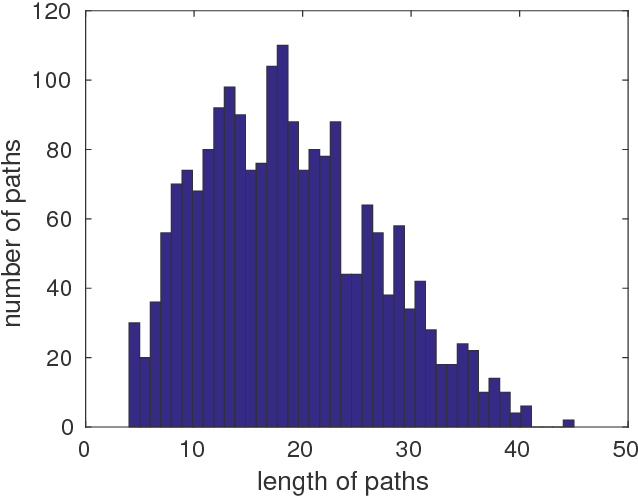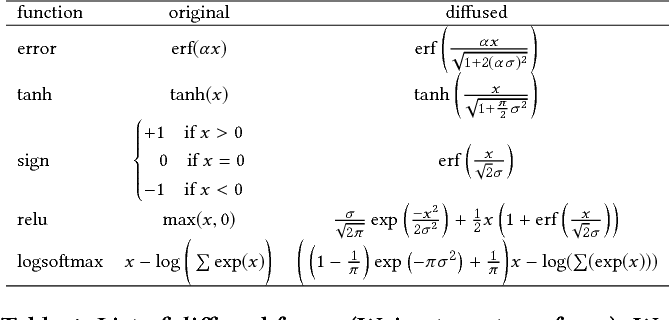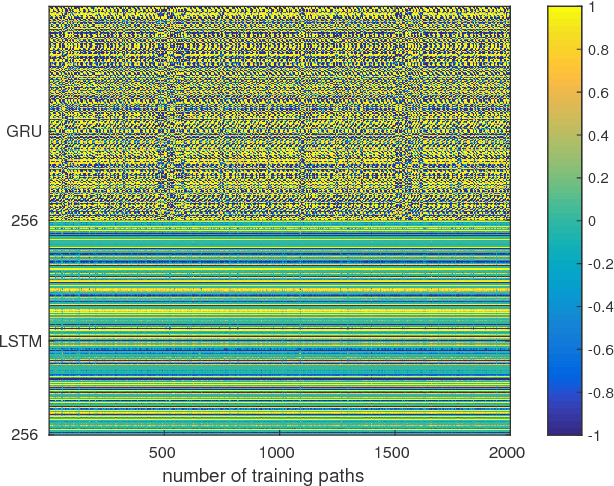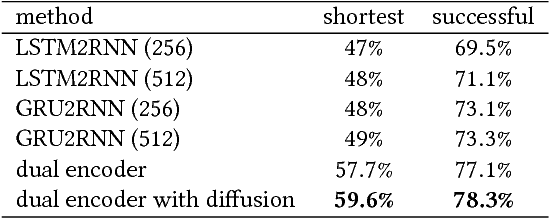A widely studied non-deterministic polynomial time (NP) hard problem lies in finding a route between the two nodes of a graph. Often meta-heuristics algorithms such as $A^{*}$ are employed on graphs with a large number of nodes. Here, we propose a deep recurrent neural network architecture based on the Sequence-2-Sequence (Seq2Seq) model, widely used, for instance in text translation. Particularly, we illustrate that utilising a context vector that has been learned from two different recurrent networks enables increased accuracies in learning the shortest route of a graph. Additionally, we show that one can boost the performance of the Seq2Seq network by smoothing the loss function using a homotopy continuation of the decoder's loss function.

Click to Read Paper and Get Code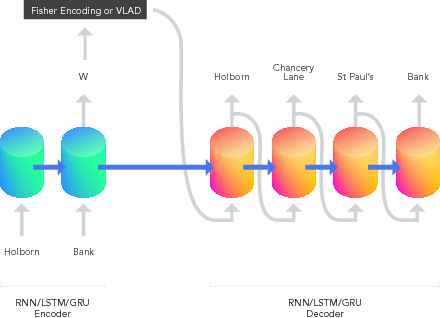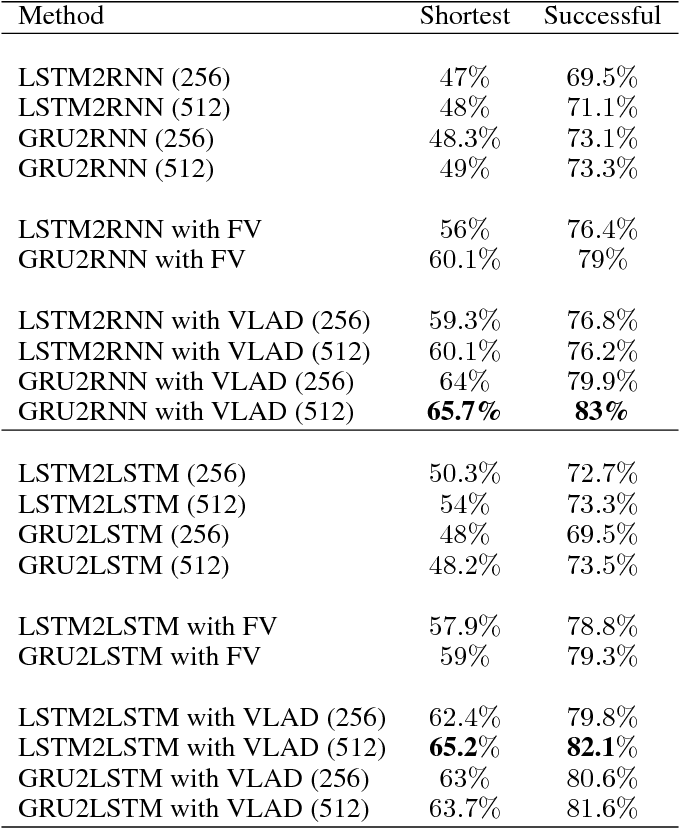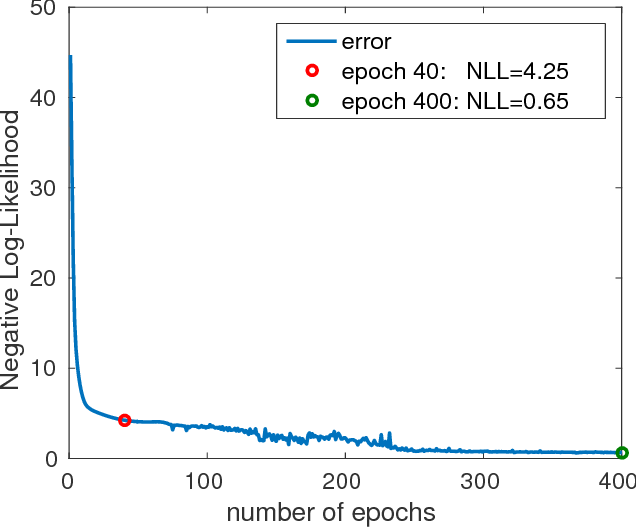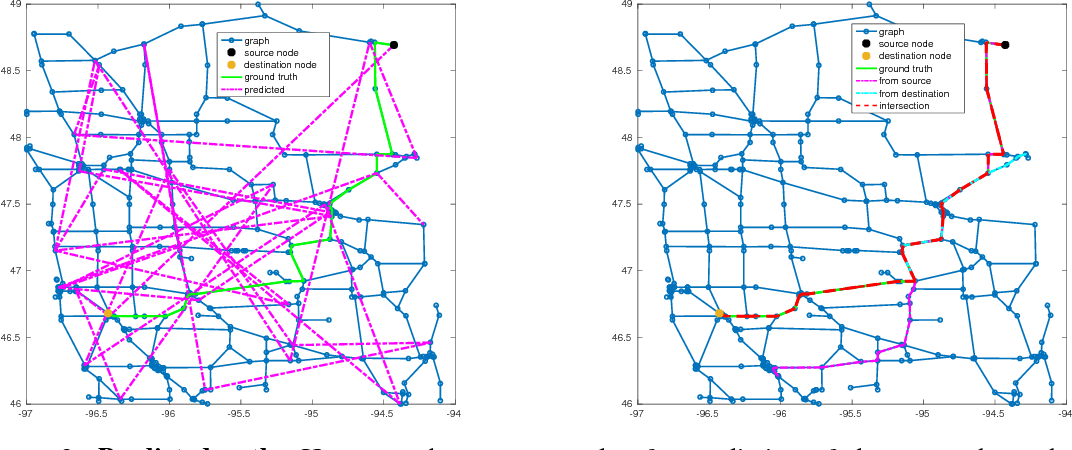The Fisher information metric is an important foundation of information geometry, wherein it allows us to approximate the local geometry of a probability distribution. Recurrent neural networks such as the Sequence-to-Sequence (Seq2Seq) networks that have lately been used to yield state-of-the-art performance on speech translation or image captioning have so far ignored the geometry of the latent embedding, that they iteratively learn. We propose the information geometric Seq2Seq (GeoSeq2Seq) network which abridges the gap between deep recurrent neural networks and information geometry. Specifically, the latent embedding offered by a recurrent network is encoded as a Fisher kernel of a parametric Gaussian Mixture Model, a formalism common in computer vision. We utilise such a network to predict the shortest routes between two nodes of a graph by learning the adjacency matrix using the GeoSeq2Seq formalism; our results show that for such a problem the probabilistic representation of the latent embedding supersedes the non-probabilistic embedding by 10-15\%.

Click to Read Paper and Get Code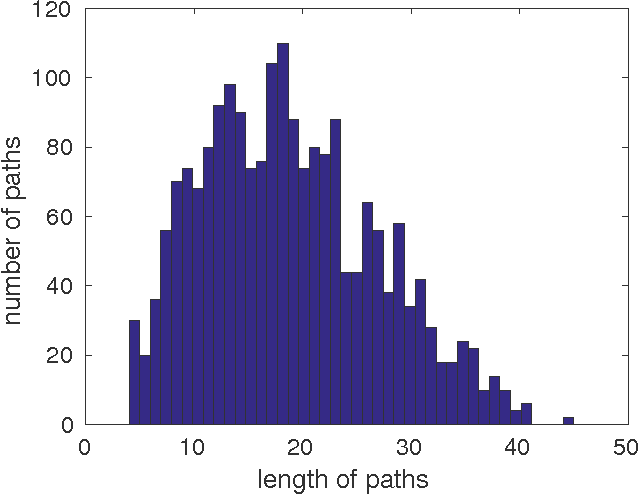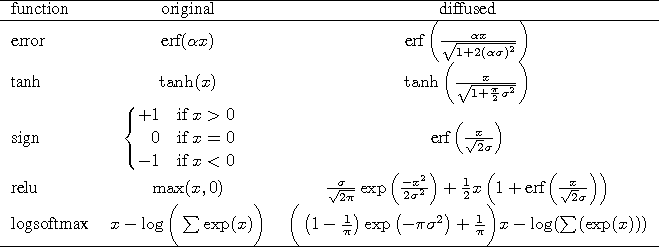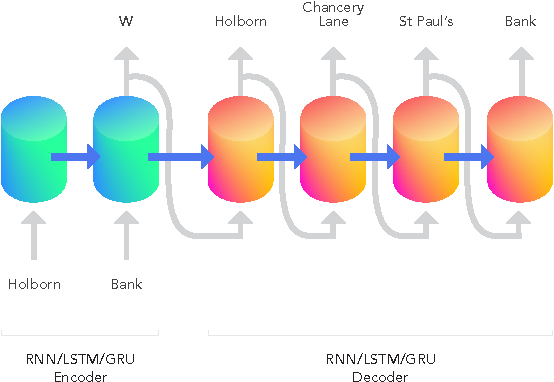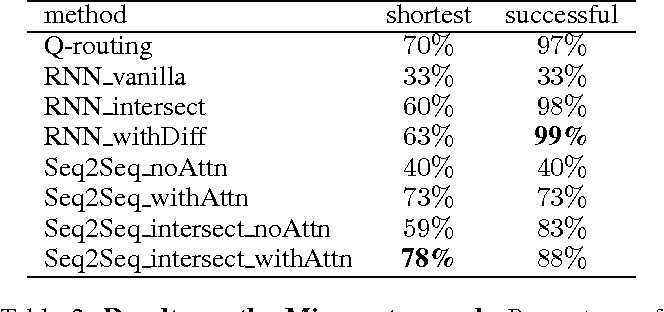Much combinatorial optimisation problems constitute a non-polynomial (NP) hard optimisation problem, i.e., they can not be solved in polynomial time. One such problem is finding the shortest route between two nodes on a graph. Meta-heuristic algorithms such as $A^{*}$ along with mixed-integer programming (MIP) methods are often employed for these problems. Our work demonstrates that it is possible to approximate solutions generated by a meta-heuristic algorithm using a deep recurrent neural network. We compare different methodologies based on reinforcement learning (RL) and recurrent neural networks (RNN) to gauge their respective quality of approximation. We show the viability of recurrent neural network solutions on a graph that has over 300 nodes and argue that a sequence-to-sequence network rather than other recurrent networks has improved approximation quality. Additionally, we argue that homotopy continuation -- that increases chances of hitting an extremum -- further improves the estimate generated by a vanilla RNN.

Click to Read Paper and Get Code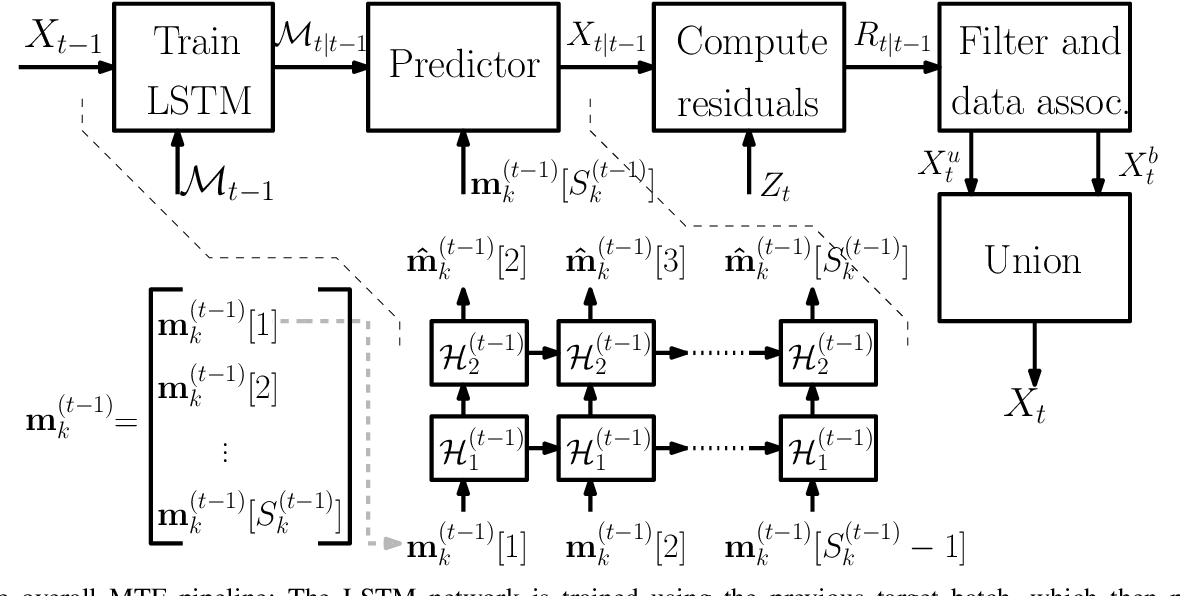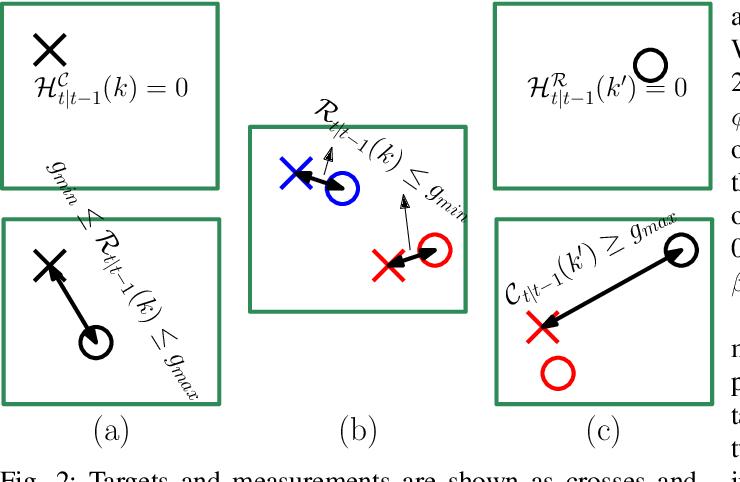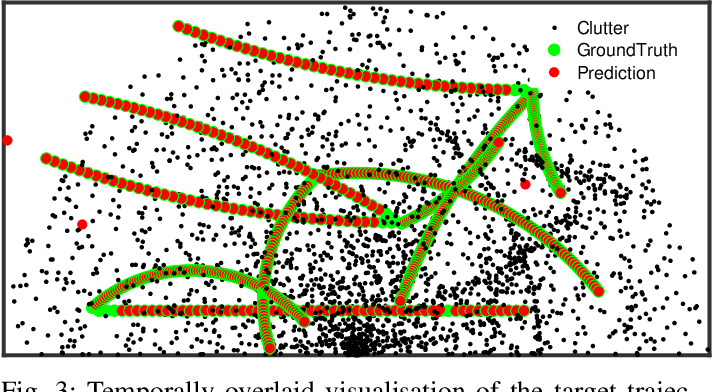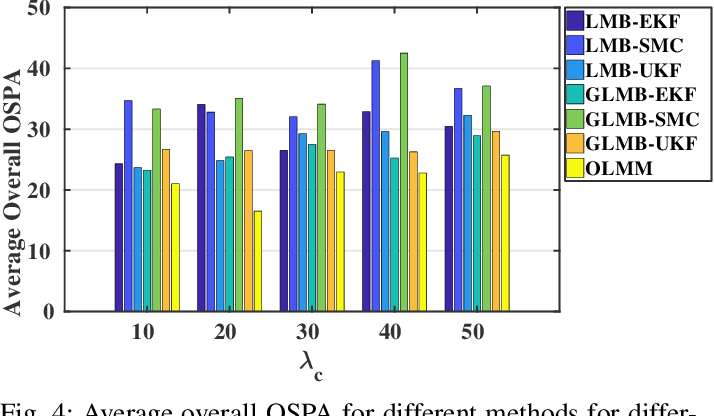Filtering point targets in highly cluttered and noisy data frames can be very challenging, especially for complex target motions. Fixed motion models can fail to provide accurate predictions, while learning based algorithm can be difficult to design (due to the variable number of targets), slow to train and dependent on separate train/test steps. To address these issues, this paper proposes a multi-target filtering algorithm which learns the motion models, on the fly, using a recurrent neural network with a long short-term memory architecture, as a regression block. The target state predictions are then corrected using a novel data association algorithm, with a low computational complexity. The proposed algorithm is evaluated over synthetic and real point target filtering scenarios, demonstrating a remarkable performance over highly cluttered data sequences.

* arXiv admin note: text overlap with arXiv:1806.06594
Click to Read Paper and Get Code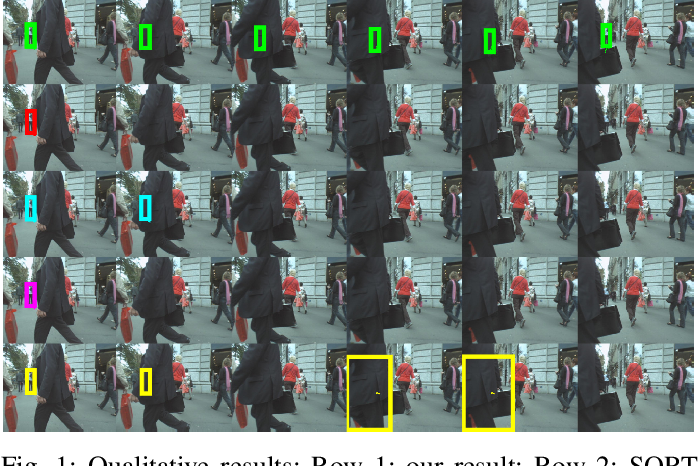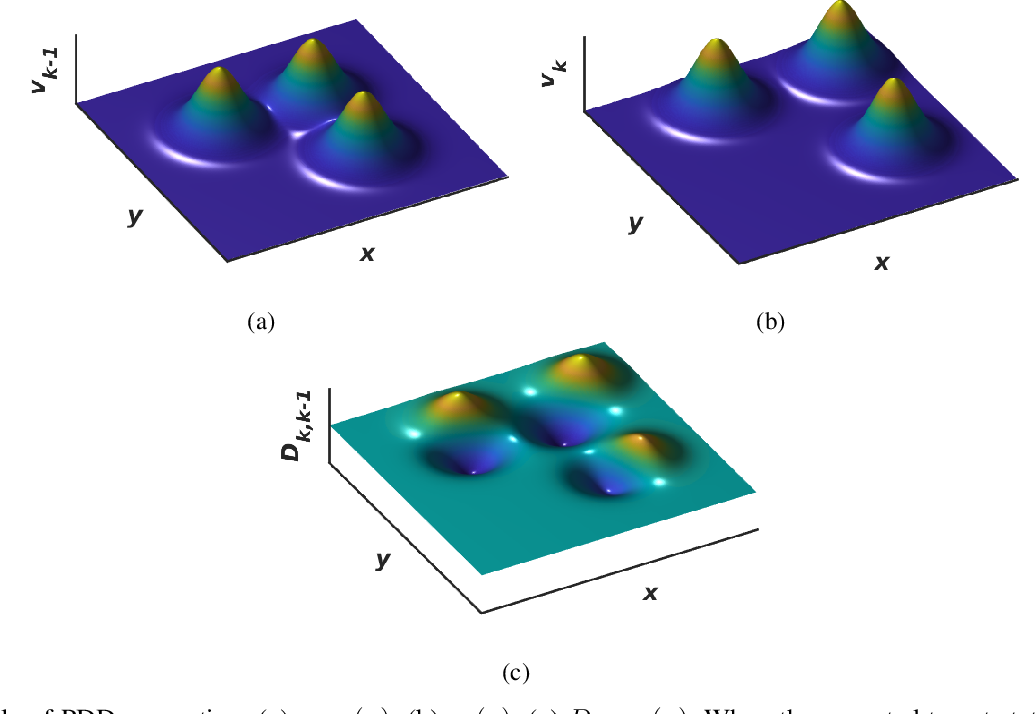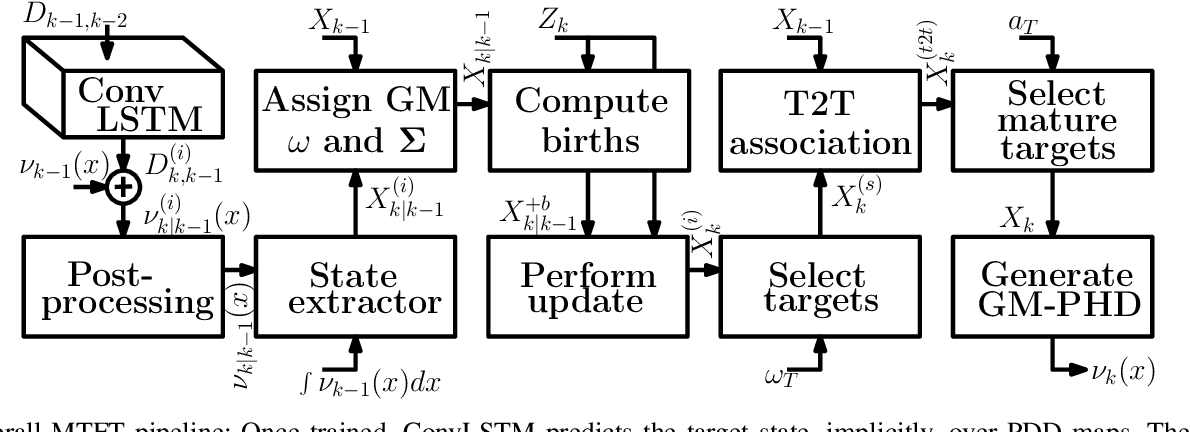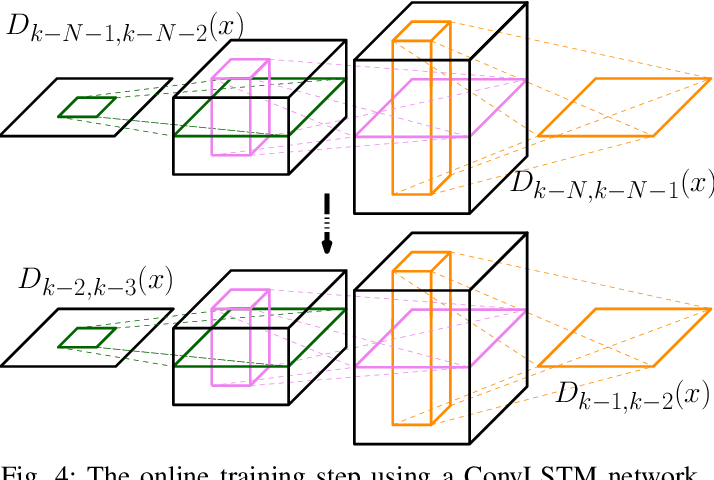Defining a multi-target motion model, which is an important step of tracking algorithms, can be very challenging. Using fixed models (as in several generative Bayesian algorithms, such as Kalman filters) can fail to accurately predict sophisticated target motions. On the other hand, sequential learning of the motion model (for example, using recurrent neural networks) can be computationally complex and difficult due to the variable unknown number of targets. In this paper, we propose a multi-target filtering and tracking (MTFT) algorithm which learns the motion model, simultaneously for all targets, from an implicitly represented state map and performs spatio-temporal data prediction. To this end, the multi-target state is modelled over a continuous hypothetical target space, using random finite sets and Gaussian mixture probability hypothesis density formulations. The prediction step is recursively performed using a deep convolutional recurrent neural network with a long short-term memory architecture, which is trained as a regression block, on the fly, over "probability density difference" maps. Our approach is evaluated over widely used pedestrian tracking benchmarks, remarkably outperforming state-of-the-art multi-target filtering algorithms, while giving competitive results when compared with other tracking approaches.

Click to Read Paper and Get Code
This paper addresses the problem of fixed motion and measurement models for multi-target filtering using an adaptive learning framework. This is performed by defining target tuples with random finite set terminology and utilisation of recurrent neural networks with a long short-term memory architecture. A novel data association algorithm compatible with the predicted tracklet tuples is proposed, enabling the update of occluded targets, in addition to assigning birth, survival and death of targets. The algorithm is evaluated over a commonly used filtering simulation scenario, with highly promising results.

* The 25th International Conference on MultiMedia Modeling (MMM)
Click to Read Paper and Get Code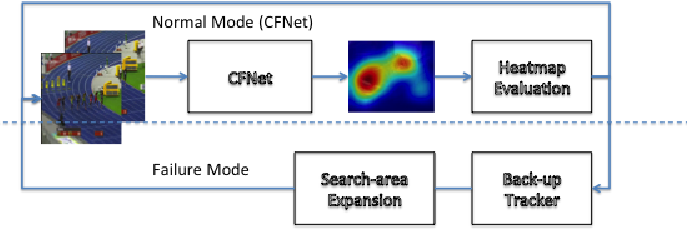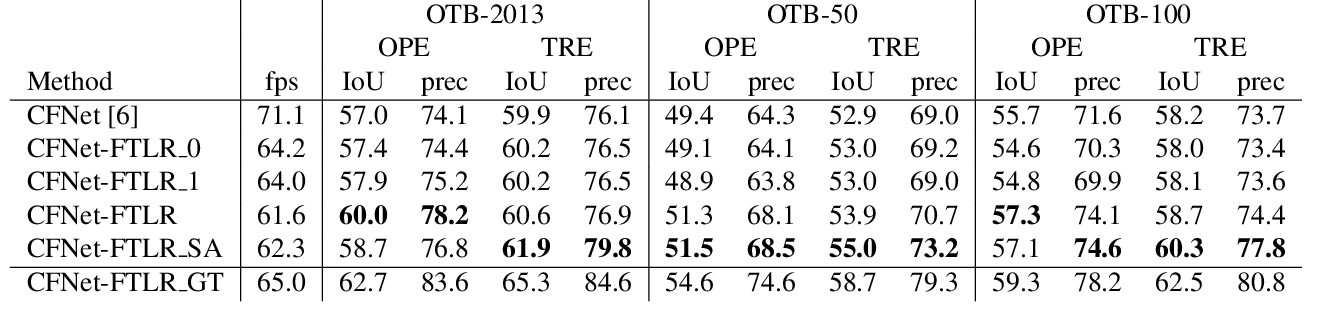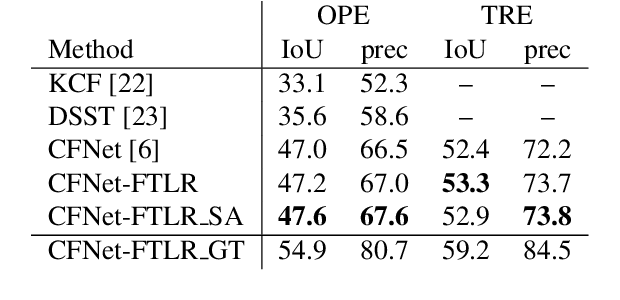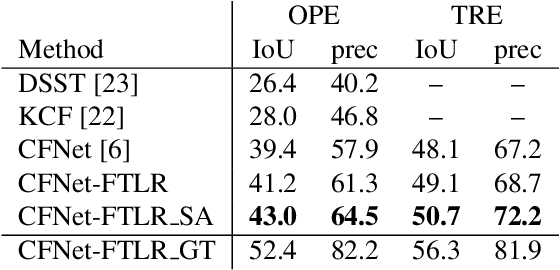In this paper, we introduce a variation of a state-of-the-art real-time tracker (CFNet), which adds to the original algorithm robustness to target loss without a significant computational overhead. The new method is based on the assumption that the feature map can be used to estimate the tracking confidence more accurately. When the confidence is low, we avoid updating the object's position through the feature map; instead, the tracker passes to a single-frame failure mode, during which the patch's low-level visual content is used to swiftly update the object's position, before recovering from the target loss in the next frame. The experimental evidence provided by evaluating the method on several tracking datasets validates both the theoretical assumption that the feature map is associated to tracking confidence, and that the proposed implementation can achieve target recovery in multiple scenarios, without compromising the real-time performance.

* arXiv admin note: substantial text overlap with arXiv:1806.07844
Click to Read Paper and Get Code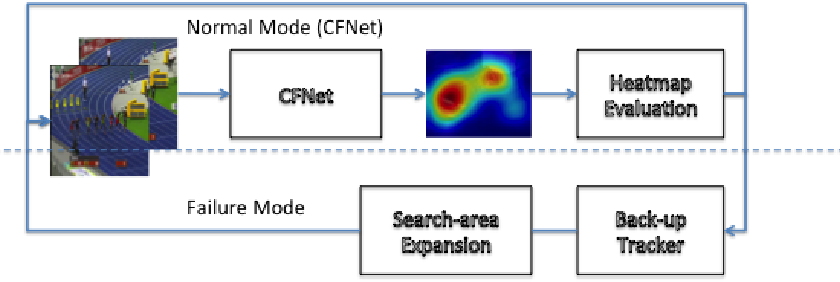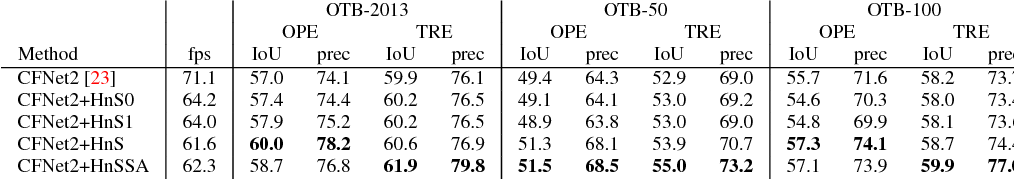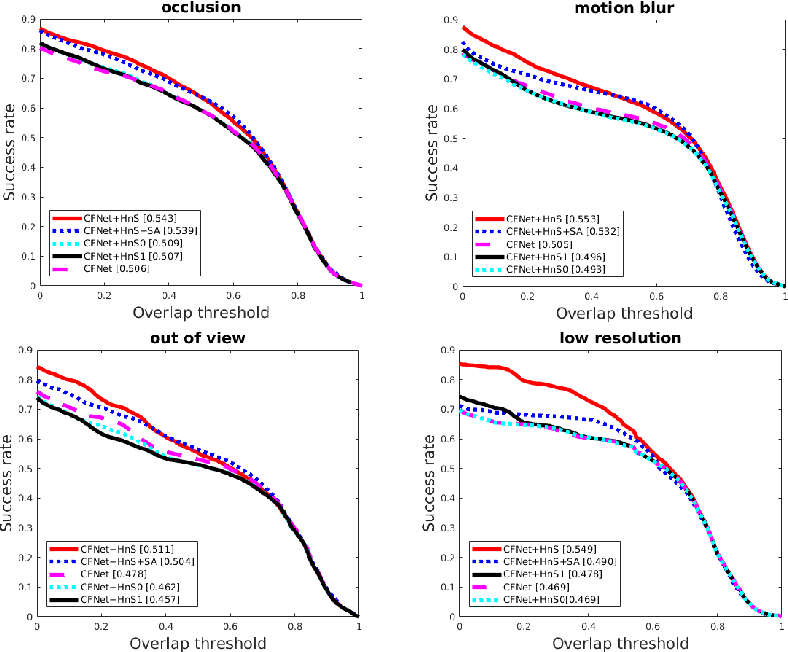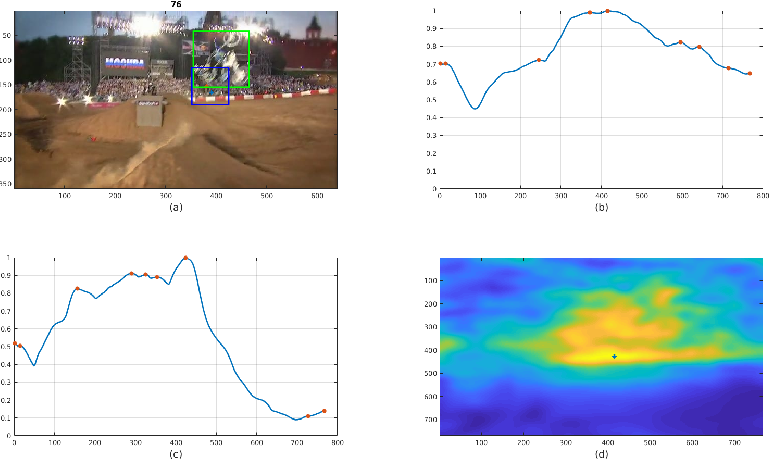In this paper, we examine the real-time recovery of a video tracker from a target loss, using information that is already available from the original tracker and without a significant computational overhead. More specifically, before using the tracker output to update the target position we estimate the detection confidence. In the case of a low confidence, the position update is rejected and the tracker passes to a single-frame failure mode, during which the patch low-level visual content is used to swiftly update the object position, before recovering from the target loss in the next frame. Orthogonally to this improvement, we further enhance the running average method used for creating the query model in tracking-through-similarity. The experimental evidence provided by evaluation on standard tracking datasets (OTB-50, OTB-100 and OTB-2013) validate that target recovery can be successfully achieved without compromising the real-time update of the target position.

Click to Read Paper and Get Code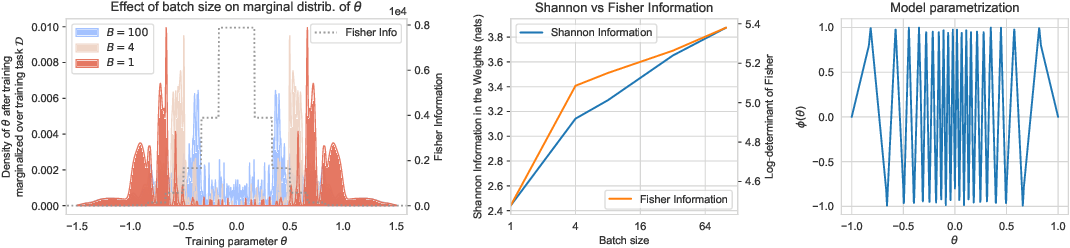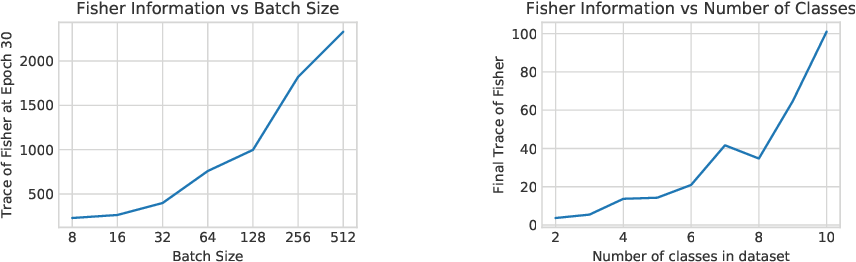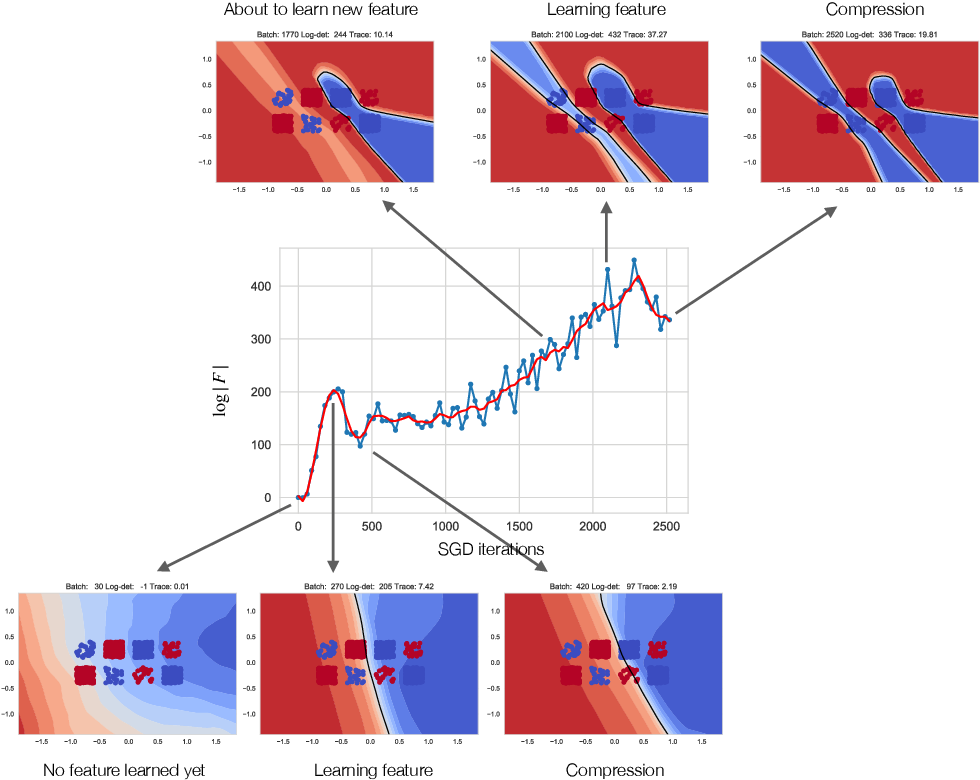Whatever information a Deep Neural Network has gleaned from past data is encoded in its weights. How this information affects the response of the network to future data is largely an open question. In fact, even how to define and measure information in a network is still not settled. We introduce the notion of Information in the Weights as the optimal trade-off between accuracy of the network and complexity of the weights, relative to a prior. Depending on the prior, the definition reduces to known information measures such as Shannon Mutual Information and Fisher Information, but affords added flexibility that enables us to relate it to generalization, via the PAC-Bayes bound, and to invariance. This relation hinges not only on the architecture of the model, but surprisingly on how it is trained. We then introduce a notion of effective information in the activations, which are deterministic functions of future inputs, resolving inconsistencies in prior work. We relate this to the Information in the Weights, and use this result to show that models of low complexity not only generalize better, but are bound to learn invariant representations of future inputs.

Click to Read Paper and Get Code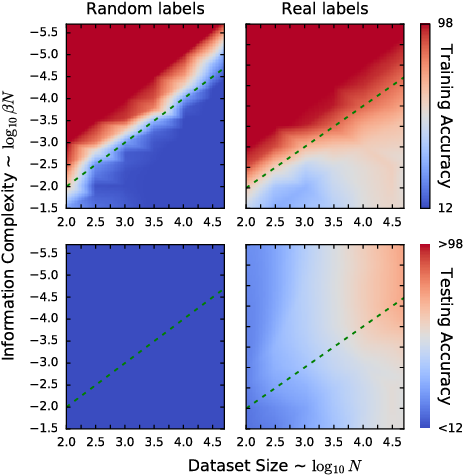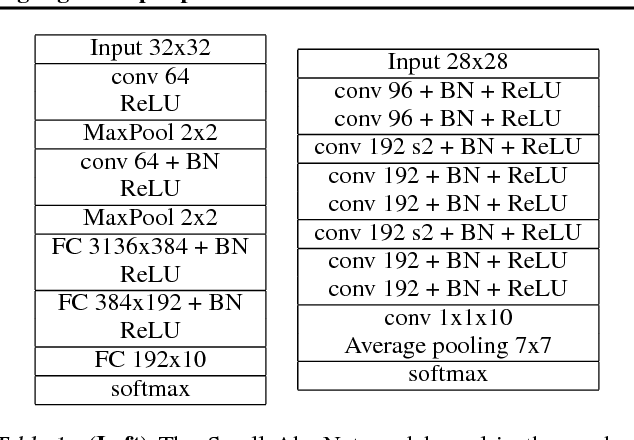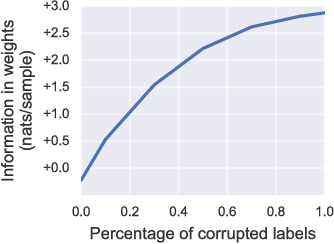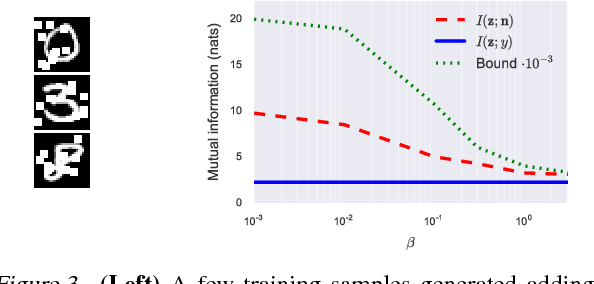Using established principles from Statistics and Information Theory, we show that invariance to nuisance factors in a deep neural network is equivalent to information minimality of the learned representation, and that stacking layers and injecting noise during training naturally bias the network towards learning invariant representations. We then decompose the cross-entropy loss used during training and highlight the presence of an inherent overfitting term. We propose regularizing the loss by bounding such a term in two equivalent ways: One with a Kullbach-Leibler term, which relates to a PAC-Bayes perspective; the other using the information in the weights as a measure of complexity of a learned model, yielding a novel Information Bottleneck for the weights. Finally, we show that invariance and independence of the components of the representation learned by the network are bounded above and below by the information in the weights, and therefore are implicitly optimized during training. The theory enables us to quantify and predict sharp phase transitions between underfitting and overfitting of random labels when using our regularized loss, which we verify in experiments, and sheds light on the relation between the geometry of the loss function, invariance properties of the learned representation, and generalization error.

* Deep learning, neural network, representation, flat minima, information bottleneck, overfitting, generalization, sufficiency, minimality, sensitivity, information complexity, stochastic gradient descent, regularization, total correlation, PAC-Bayes
Click to Read Paper and Get Code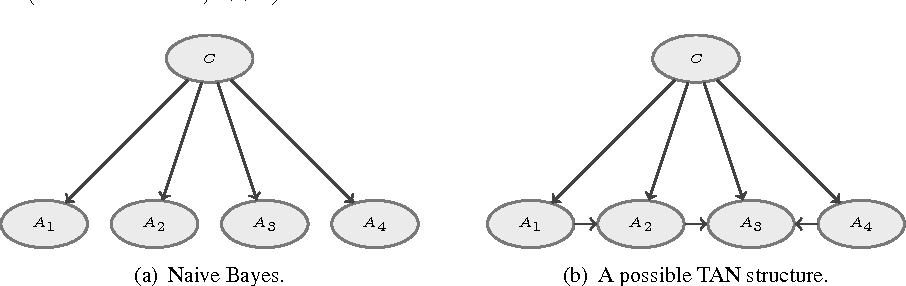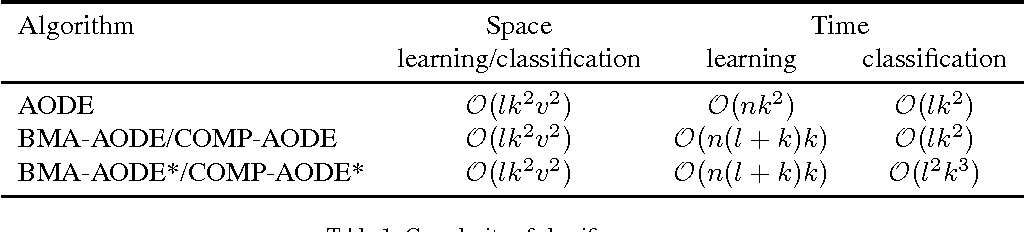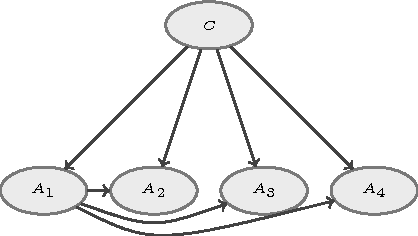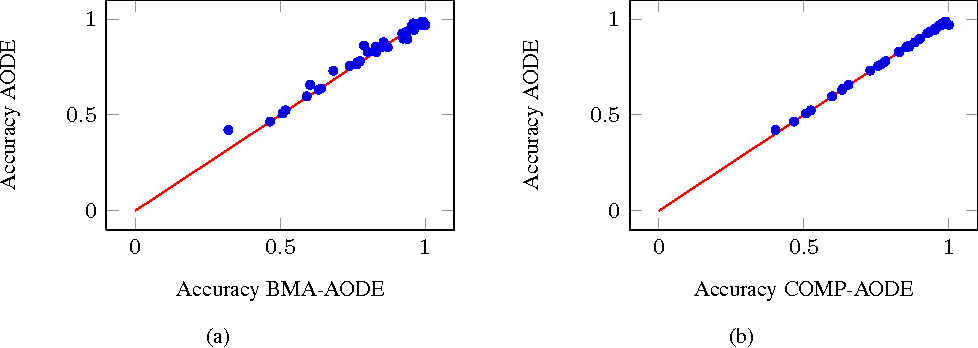Bayesian model averaging (BMA) is an approach to average over alternative models; yet, it usually gets excessively concentrated around the single most probable model, therefore achieving only sub-optimal classification performance. The compression-based approach (Boulle, 2007) overcomes this problem, averaging over the different models by applying a logarithmic smoothing over the models' posterior probabilities. This approach has shown excellent performances when applied to ensembles of naive Bayes classifiers. AODE is another ensemble of models with high performance (Webb, 2005), based on a collection of non-naive classifiers (called SPODE) whose probabilistic predictions are aggregated by simple arithmetic mean. Aggregating the SPODEs via BMA rather than by arithmetic mean deteriorates the performance; instead, we aggregate the SPODEs via the compression coefficients and we show that the resulting classifier obtains a slight but consistent improvement over AODE. However, an important issue in any Bayesian ensemble of models is the arbitrariness in the choice of the prior over the models. We address this problem by the paradigm of credal classification, namely by substituting the unique prior with a set of priors. Credal classifier automatically recognize the prior-dependent instances, namely the instances whose most probable class varies, when different priors are considered; in these cases, credal classifiers remain reliable by returning a set of classes rather than a single class. We thus develop the credal version of both the BMA-based and the compression-based ensemble of SPODEs, substituting the single prior over the models by a set of priors. Experiments show that both credal classifiers provide higher classification reliability than their determinate counterparts; moreover the compression-based credal classifier compares favorably to previous credal classifiers.

Click to Read Paper and Get Code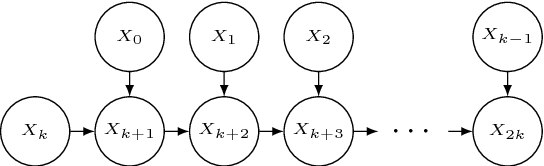A reliable modeling of uncertain evidence in Bayesian networks based on a set-valued quantification is proposed. Both soft and virtual evidences are considered. We show that evidence propagation in this setup can be reduced to standard updating in an augmented credal network, equivalent to a set of consistent Bayesian networks. A characterization of the computational complexity for this task is derived together with an efficient exact procedure for a subclass of instances. In the case of multiple uncertain evidences over the same variable, the proposed procedure can provide a set-valued version of the geometric approach to opinion pooling.

* 19 pages
Click to Read Paper and Get Code
We review the problem of defining and inferring a "state" for a control system based on complex, high-dimensional, highly uncertain measurement streams such as videos. Such a state, or representation, should contain all and only the information needed for control, and discount nuisance variability in the data. It should also have finite complexity, ideally modulated depending on available resources. This representation is what we want to store in memory in lieu of the data, as it "separates" the control task from the measurement process. For the trivial case with no dynamics, a representation can be inferred by minimizing the Information Bottleneck Lagrangian in a function class realized by deep neural networks. The resulting representation has much higher dimension than the data, already in the millions, but it is smaller in the sense of information content, retaining only what is needed for the task. This process also yields representations that are invariant to nuisance factors and having maximally independent components. We extend these ideas to the dynamic case, where the representation is the posterior density of the task variable given the measurements up to the current time, which is in general much simpler than the prediction density maintained by the classical Bayesian filter. Again this can be finitely-parametrized using a deep neural network, and already some applications are beginning to emerge. No explicit assumption of Markovianity is needed; instead, complexity trades off approximation of an optimal representation, including the degree of Markovianity.

Click to Read Paper and Get Code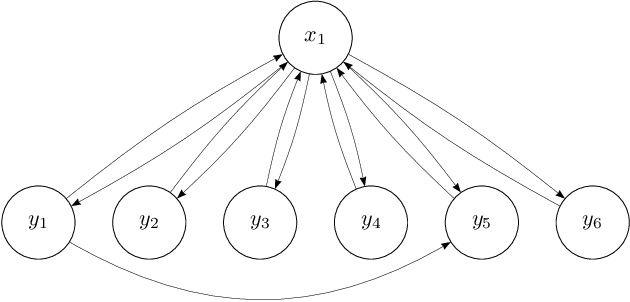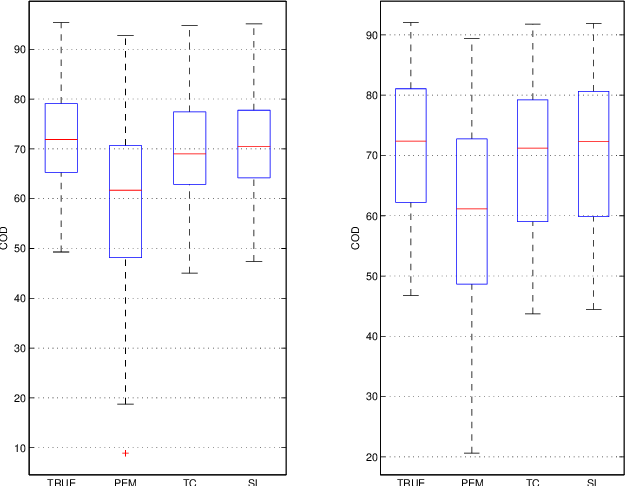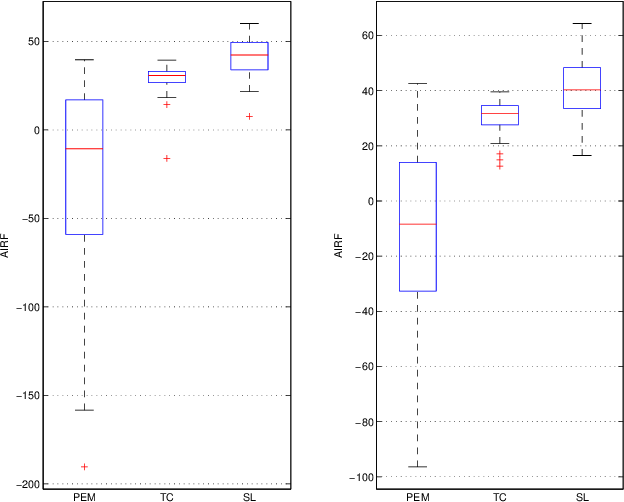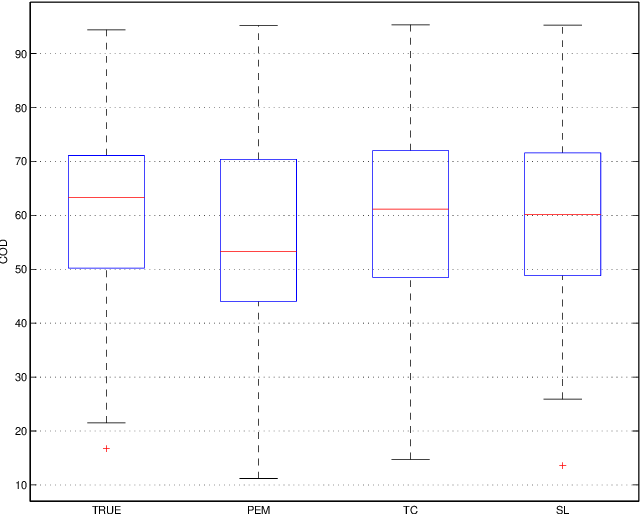We consider the problem of modeling multivariate time series with parsimonious dynamical models which can be represented as sparse dynamic Bayesian networks with few latent nodes. This structure translates into a sparse plus low rank model. In this paper, we propose a Gaussian regression approach to identify such a model.

Click to Read Paper and Get Code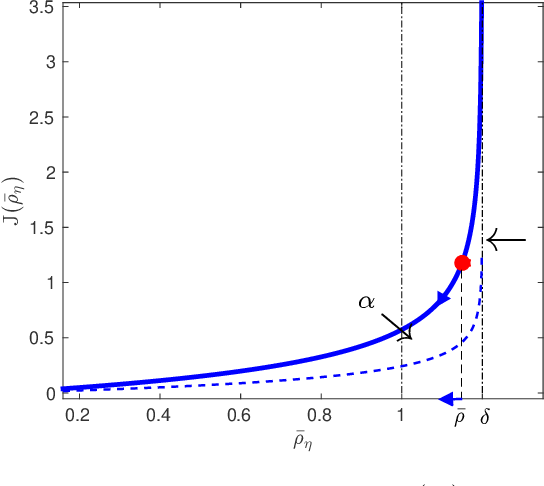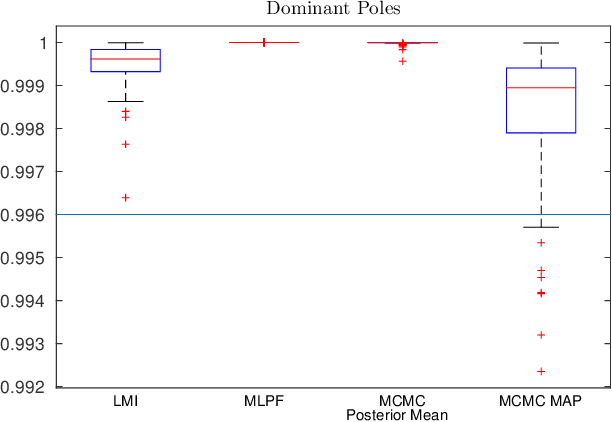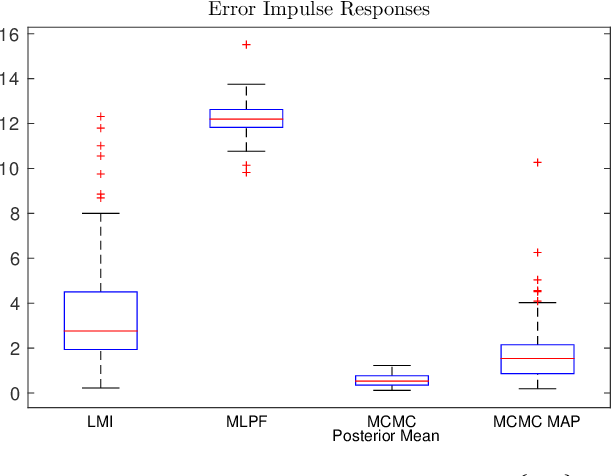A new Bayesian approach to linear system identification has been proposed in a series of recent papers. The main idea is to frame linear system identification as predictor estimation in an infinite dimensional space, with the aid of regularization/Bayesian techniques. This approach guarantees the identification of stable predictors based on the prediction error minimization. Unluckily, the stability of the predictors does not guarantee the stability of the impulse response of the system. In this paper we propose and compare various techniques to address this issue. Simulations results comparing these techniques will be provided.

* number of pages = 6, number of figures = 3
Click to Read Paper and Get Code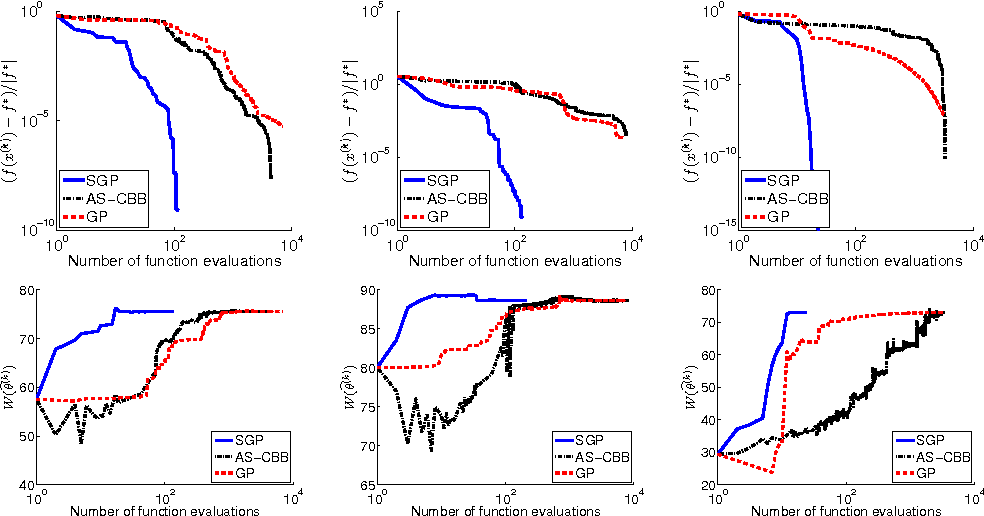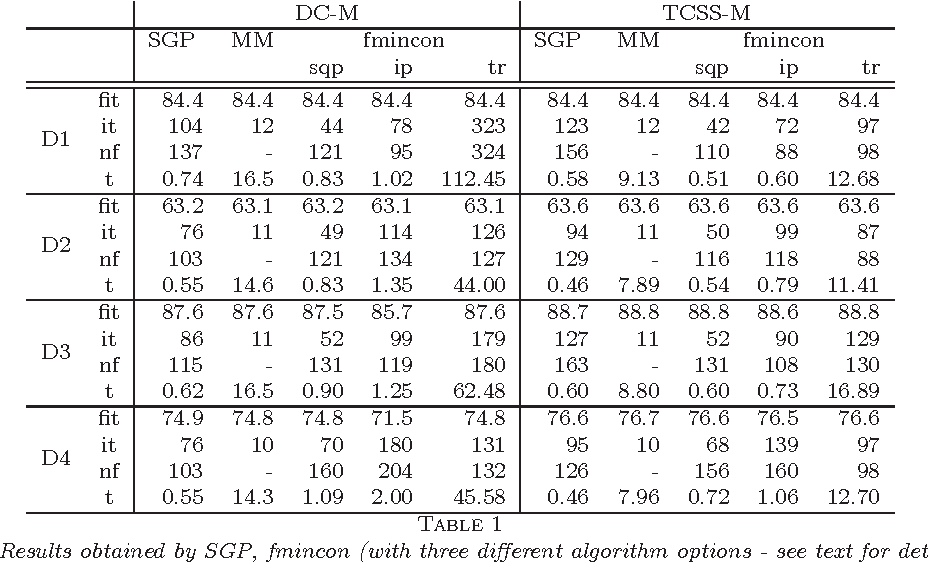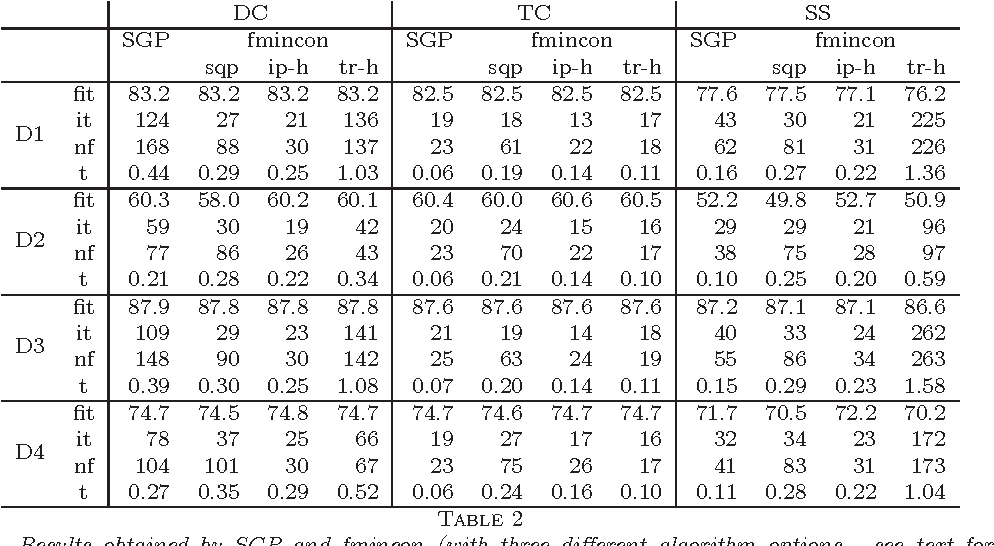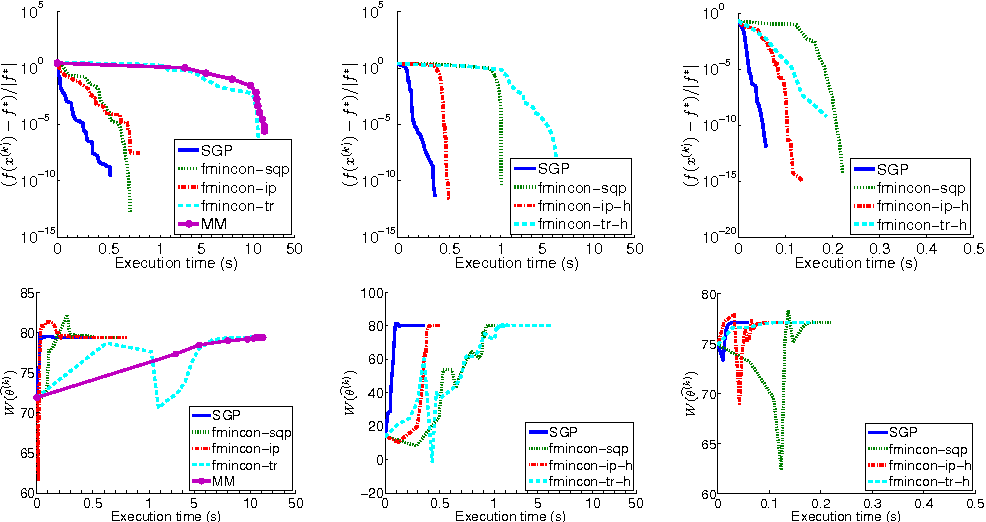A crucial task in system identification problems is the selection of the most appropriate model class, and is classically addressed resorting to cross-validation or using asymptotic arguments. As recently suggested in the literature, this can be addressed in a Bayesian framework, where model complexity is regulated by few hyperparameters, which can be estimated via marginal likelihood maximization. It is thus of primary importance to design effective optimization methods to solve the corresponding optimization problem. If the unknown impulse response is modeled as a Gaussian process with a suitable kernel, the maximization of the marginal likelihood leads to a challenging nonconvex optimization problem, which requires a stable and effective solution strategy. In this paper we address this problem by means of a scaled gradient projection algorithm, in which the scaling matrix and the steplength parameter play a crucial role to provide a meaning solution in a computational time comparable with second order methods. In particular, we propose both a generalization of the split gradient approach to design the scaling matrix in the presence of box constraints, and an effective implementation of the gradient and objective function. The extensive numerical experiments carried out on several test problems show that our method is very effective in providing in few tenths of a second solutions of the problems with accuracy comparable with state-of-the-art approaches. Moreover, the flexibility of the proposed strategy makes it easily adaptable to a wider range of problems arising in different areas of machine learning, signal processing and system identification.

* SIAM Journal on Scientific Computing 37 (2015), A1297-A1318
Click to Read Paper and Get Code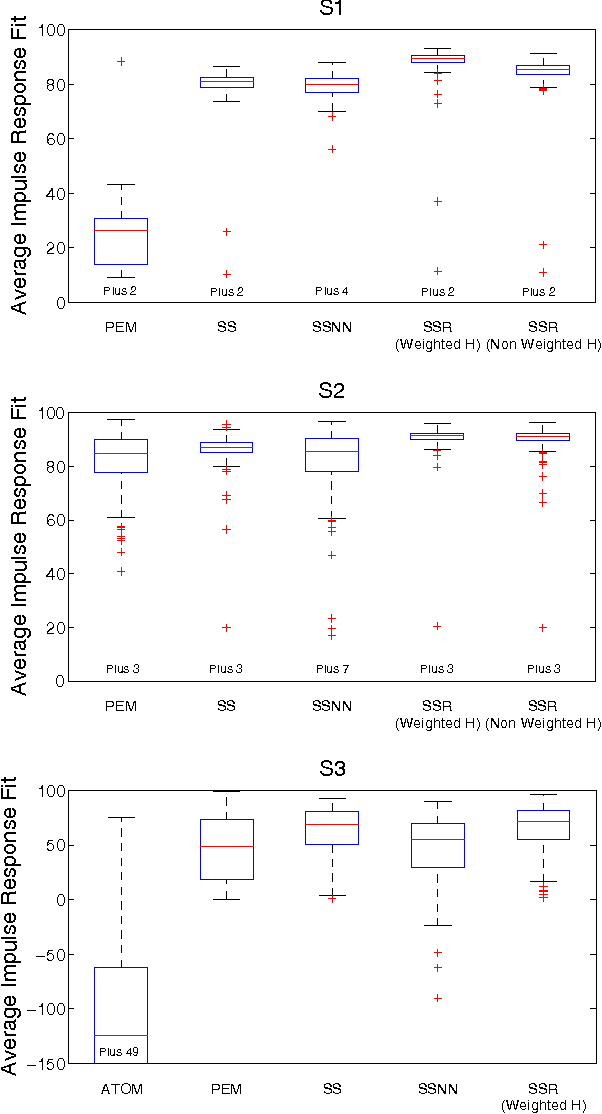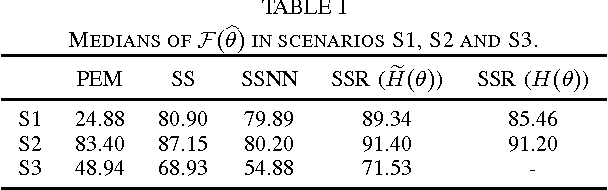Recent developments in linear system identification have proposed the use of non-parameteric methods, relying on regularization strategies, to handle the so-called bias/variance trade-off. This paper introduces an impulse response estimator which relies on an $\ell_2$-type regularization including a rank-penalty derived using the log-det heuristic as a smooth approximation to the rank function. This allows to account for different properties of the estimated impulse response (e.g. smoothness and stability) while also penalizing high-complexity models. This also allows to account and enforce coupling between different input-output channels in MIMO systems. According to the Bayesian paradigm, the parameters defining the relative weight of the two regularization terms as well as the structure of the rank penalty are estimated optimizing the marginal likelihood. Once these hyperameters have been estimated, the impulse response estimate is available in closed form. Experiments show that the proposed method is superior to the estimator relying on the "classic" $\ell_2$-regularization alone as well as those based in atomic and nuclear norm.

* to appear in IEEE Conference on Decision and Control, 2014
Click to Read Paper and Get Code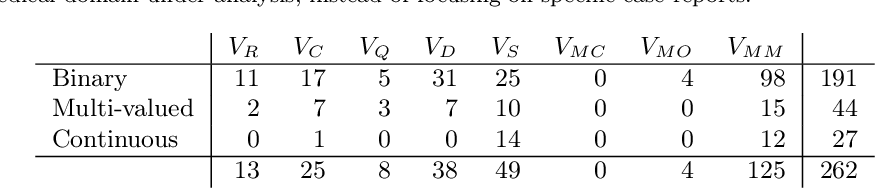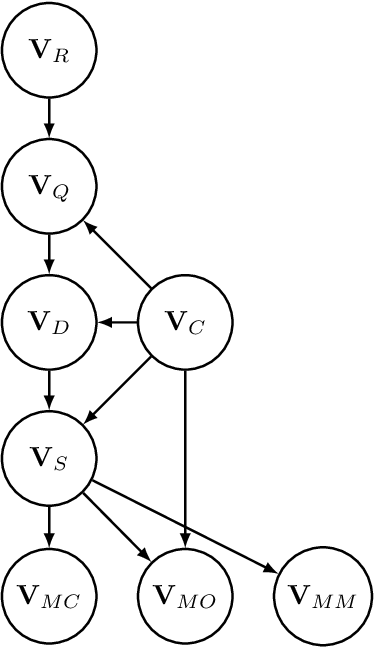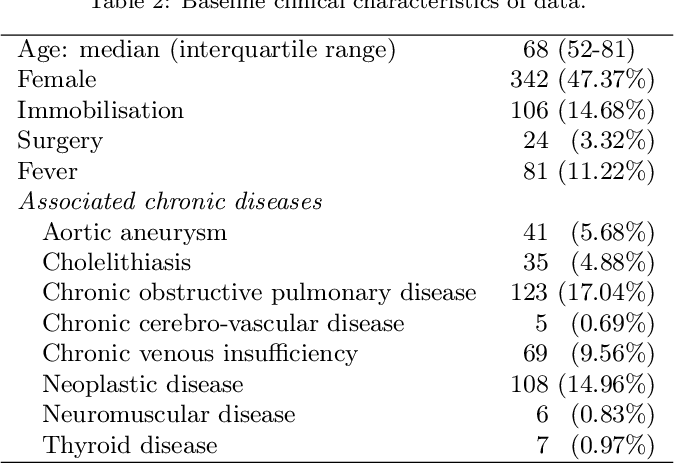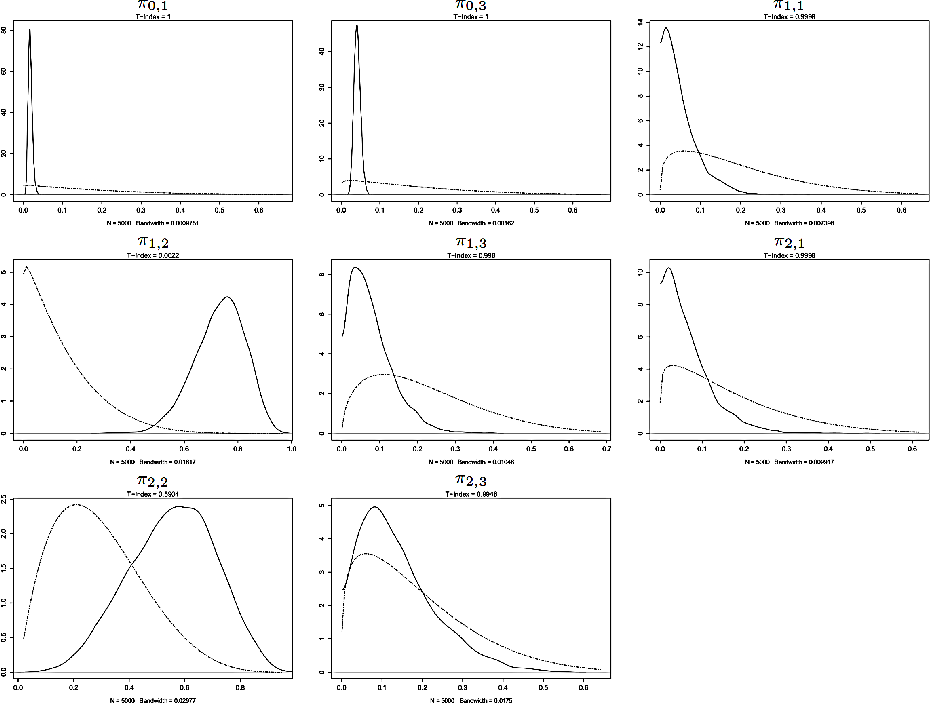In this paper, the development of a probabilistic network for the diagnosis of acute cardiopulmonary diseases is presented. This paper is a draft version of the article published after peer review in 2018 (https://doi.org/10.1002/bimj.201600206). A panel of expert physicians collaborated to specify the qualitative part, that is a directed acyclic graph defining a factorization of the joint probability distribution of domain variables. The quantitative part, that is the set of all conditional probability distributions defined by each factor, was estimated in the Bayesian paradigm: we applied a special formal representation, characterized by a low number of parameters and a parameterization intelligible for physicians, elicited the joint prior distribution of parameters from medical experts, and updated it by conditioning on a dataset of hospital patient records using Markov Chain Monte Carlo simulation. Refinement was cyclically performed until the probabilistic network provided satisfactory Concordance Index values for a selection of acute diseases and reasonable inference on six fictitious patient cases. The probabilistic network can be employed to perform medical diagnosis on a total of 63 diseases (38 acute and 25 chronic) on the basis of up to 167 patient findings.

* Biometrical Journal, 60(1), 174-195, January 2018
* The DOI of the article published after peer review was added. A technical detail was added in Section 3.2, Formula 8 (as a consequence, the ID of all the subsequent formulas result augmented by 1 with respect to the previous version). The prior standard deviation of the Gamma distribution in Table 4 was fixed (in the previous version, the prior variance was indicated, instead)
Click to Read Paper and Get Code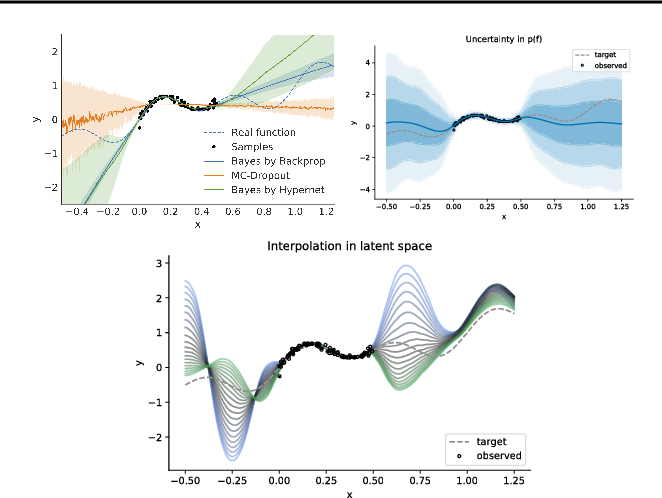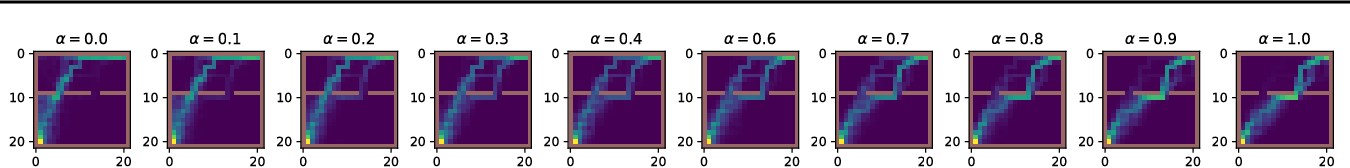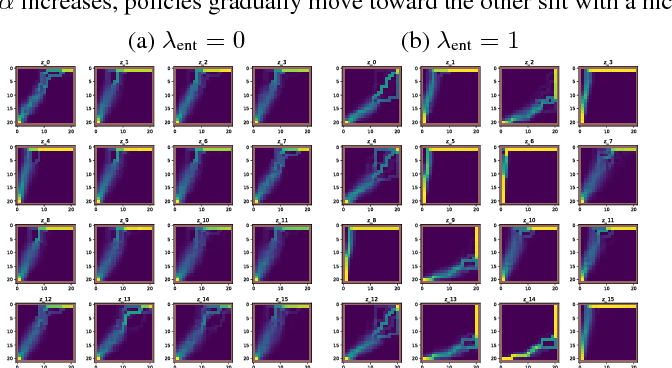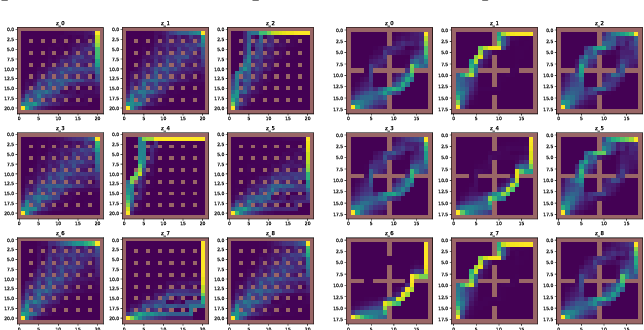We introduce a deep generative model for functions. Our model provides a joint distribution p(f, z) over functions f and latent variables z which lets us efficiently sample from the marginal p(f) and maximize a variational lower bound on the entropy H(f). We can thus maximize objectives of the form E_{f~p(f)}[R(f)] + c*H(f), where R(f) denotes, e.g., a data log-likelihood term or an expected reward. Such objectives encompass Bayesian deep learning in function space, rather than parameter space, and Bayesian deep RL with representations of uncertainty that offer benefits over bootstrapping and parameter noise. In this short paper we describe our model, situate it in the context of prior work, and present proof-of-concept experiments for regression and RL.

* To be presented at the ICML 2018 workshop on Prediction and Generative Modeling in Reinforcement Learning
Click to Read Paper and Get Code